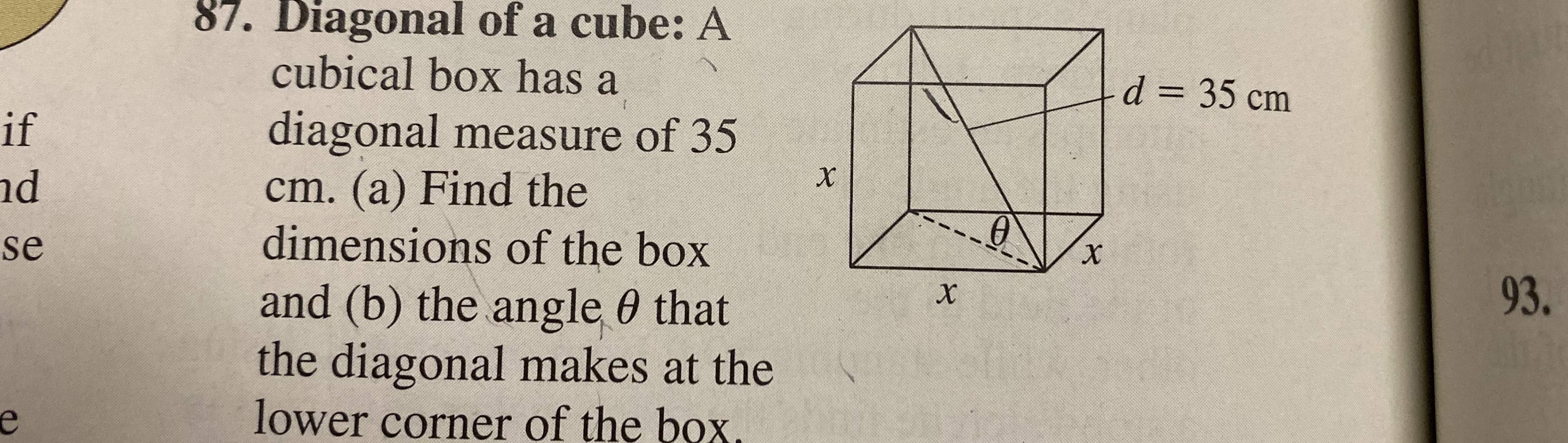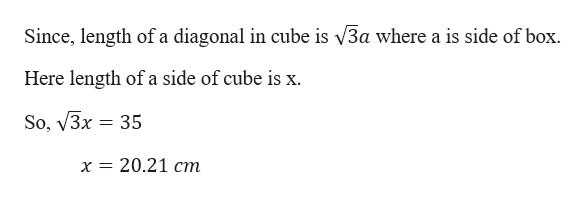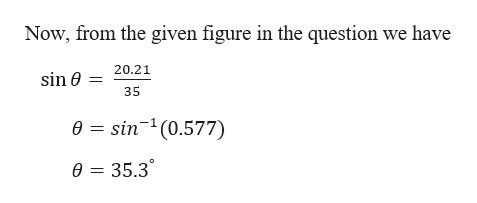# 87. Diagonal of a cube: Acubical box has adiagonal measure of 35cm. (a) Find thedimensions of the boxand (b) the angle 0 thatthe diagonal makes at thelower corner of the box.d 35 cmifdse93.

Question
4 views

Diagonal of a cube:  A cubical box has a diagonal measure of  35 cm. (a) Find the dimensions of the box. (B) the angle (theta) that the diagonal makes at the lower corner of the box.

Help. I am in Pre-Calc and I can not fiqure out the answer. I have a picture which I will add. Thank youhelp_outlineImage Transcriptionclose87. Diagonal of a cube: A cubical box has a diagonal measure of 35 cm. (a) Find the dimensions of the box and (b) the angle 0 that the diagonal makes at the lower corner of the box. d 35 cm if d se 93. fullscreen
check_circle

star
star
star
star
star
1 Rating
Step 1

Given,

A cubical box has a diagonal measure of 35 cm.

Step 2

Part ahelp_outlineImage TranscriptioncloseSince, length of a diagonal in cube is V3a where a is side of box. Here length of a side of cube is x So, V3x 35 х 3D 20.21 ст fullscreen
Step 3

Part b

...help_outlineImage TranscriptioncloseNow, from the given figure in the question we have 20.21 sin e 35 e sin(0.577) 0= 35.3 fullscreen

### Want to see the full answer?

See Solution

#### Want to see this answer and more?

Solutions are written by subject experts who are available 24/7. Questions are typically answered within 1 hour.*

See Solution
*Response times may vary by subject and question.
Tagged in

### Other